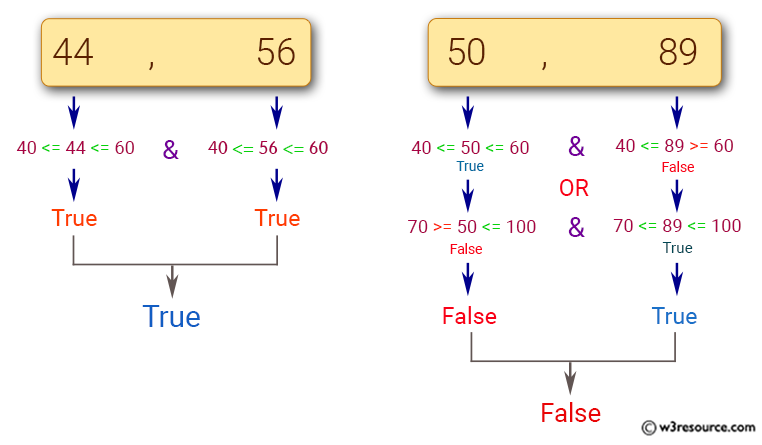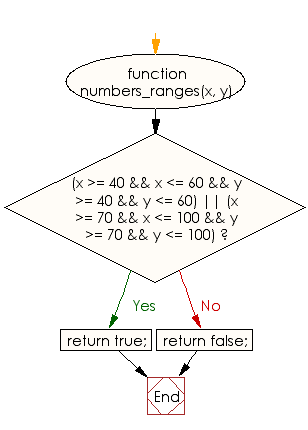# JavaScript: Check whether two numbers are in range 40..60 or in the range 70..100 inclusive

## JavaScript Basic: Exercise-33 with Solution

Write a JavaScript program to check whether two numbers are in range 40..60 or in the range 70..100 inclusive.

Pictorial Presentation:Sample Solution:

HTML Code:

``````<!DOCTYPE html>
<html>
<meta charset="utf-8">
<meta name="viewport" content="width=device-width">
<title>JavaScript program to check whether two numbers are in range 40..60 or in the range 70..100 inclusive</title>
<body>

</body>
</html>
```
```

JavaScript Code:

``````function numbers_ranges(x, y) {
if ((x >= 40 && x <= 60 && y >= 40 && y <= 60)
||
(x >= 70 && x <= 100 && y >= 70 && y <= 100))
{
return true;
}
else
{
return false;
}
}

console.log(numbers_ranges(44, 56));
console.log(numbers_ranges(70, 95));
console.log(numbers_ranges(50, 89));
```
```

Sample Output:

```true
true
false
```

Flowchart:ES6 Version:

``````function numbers_ranges(x, y) {
if ((x >= 40 && x <= 60 && y >= 40 && y <= 60)
||
(x >= 70 && x <= 100 && y >= 70 && y <= 100))
{
return true;
}
else
{
return false;
}
}

console.log(numbers_ranges(44, 56));
console.log(numbers_ranges(70, 95));
console.log(numbers_ranges(50, 89));
``````

Live Demo:

See the Pen JavaScript: check if two numbers are in a range - basic- ex-33 by w3resource (@w3resource) on CodePen.

Improve this sample solution and post your code through Disqus

What is the difficulty level of this exercise?

Test your Programming skills with w3resource's quiz.

﻿

## JavaScript: Tips of the Day

Checks if a string is an anagram of another string (case-insensitive, ignores spaces, punctuation and special characters)

Example:

```const isAnagram = (str1, str2) => {
const normalize = str =>
str
.toLowerCase()
.replace(/[^a-z0-9]/gi, '')
.split('')
.sort()
.join('');
return normalize(str1) === normalize(str2);
};
console.log(isAnagram('iceman', 'cinema')); // true
```

Output:

```true
```